# Triangle

A polygon or 2-dimensional plane figure having sides, vertex and angles all three in number is said to be a triangle. We are much aware of a triangle, its type and some properties related to it.

Let’s revise them:

There are 3 types of triangle mainly on basis of sides:
1. Equilateral Triangle: All sides and angles are equal and angle equal to 60⁰.
2. Isosceles Triangle: Two sides are equal and angle adjacent to it are also equal.
3. Scalene triangle: No side, as well as angles, are equal.

There are 3 types of triangle mainly on basis of internal angle:
1
.Right triangle: A triangle in which one of the internal
angle measures 90⁰.

In this triangle, the largest of the three sides which lies opposite to the right angle is called hypotenuse.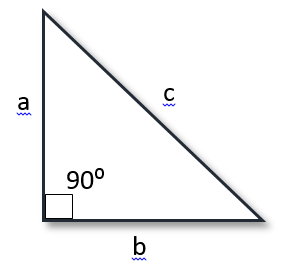Pythagorean Theorem: The sum of squares of the adjacent sides attached to the right angle is equal to the square of hypotenuse.
i.e. a2 + b2 = c2

1. Acute Triangle: When internal angle of a triangle has measure of less than 90⁰, it is said to be acute triangle.
2. Obtuse Triangle: When an internal angle of a triangle has measure of more than 90⁰, it is said to be obtuse triangle.

## Congruence of Figures:

Before coming to triangles let’s first know about what exactly the congruent figure is?

Two figures either plane or 3 dimensional having exactly same shape and size so that one figure is exactly fitted to other, then these figures are said to be congruent.

Example:

• Coins of one rupee are congruent.
• Circles with same radii are congruent.
• Squares of same side are congruent.

## Congruence of triangles:

Two triangles are said to be congruent if all the three corresponding sides, as well as angles of the one, exactly matches with the other.

For Mathematical purpose to determine congruence of triangle there is some proved rule so that one can easily identify whether the figures are congruent or not.

## Criteria for Congruence of Triangles:

1. SAS Congruence Rule:

SAS stands for Side – Angle – Side.

• Two triangles are said to be congruent according to SAS rule if two sides and the included angle of one triangle are equal to the sides and the included angle of the other triangle.1. ASA congruence rule :

ASA stands for Angle – Side – Angle

• Two triangles are congruent if two angles and the included side of one triangle are equal to two angles and the included side of other triangle.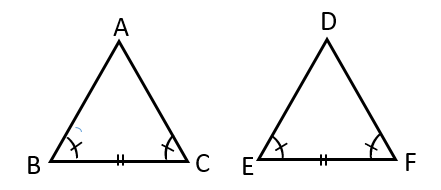By this figure we can say that ΔABC ≅ ΔDEF (By ASA rule of congruency)

3. SSS congruence rule:

SSS stands for Side – Side – Side

• If all the three sides of one triangle is exactly equal to three sides of the other triangle than this rule of comparison is said to be SSS congruence rule.In ΔABC and ΔDEF, AB=DE, BC=EF, AC=DF thus congruence according to SSS rule.

1. RHS Congruence rule:

RHS stands for Right Hypotenuse Side.

• If we compare two right triangles and the Hypotenuse and any one of the sides of one triangle is equal to the other triangle, then both triangle are said to be congruent by RHS congruence rule.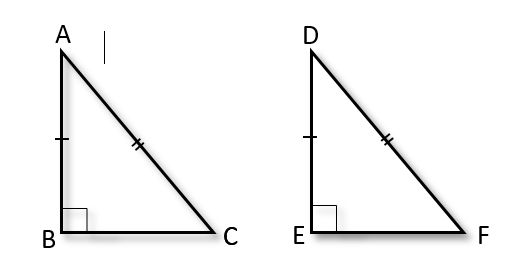In ΔABC and ΔDEF, AB=DE, AC=DF (hypotenuse) thus congruence according to RHS rule.

## Properties of Triangles:

• In an isosceles triangle angle opposite to equal sides are also equal in measure.
• In an isosceles triangle sides opposite to equal angles are also equal in measure.
• If in a triangle two sides of are unequal, the angle opposite to thelonger side is larger (or greater).
• In a triangle the side opposite to the greatest (larger) angle is larger of three sides.
• In any triangle, sum of any two sides is always greater than the third side.

## Examples related to Congruence of Triangles:

1. In figure, If OA = OB and OD = OC. Show that Δ AOD Δ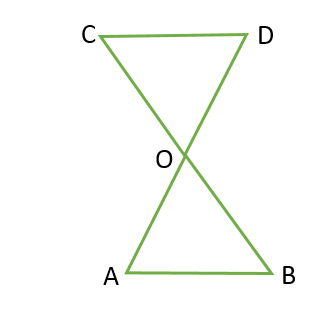Solution:

InΔ AOD and Δ BOC,
Given,
OA = OB
OD = OC
Also, since ∠ AOD and ∠ BOC form a pair of vertically opposite angles,
we have
∠ AOD = ∠ BOC.

So, Δ AOD ≅ Δ BOC (by the SAS congruence rule)

1. In given figure Line-segment AB is parallel to another line-segment CD. O is the mid-point of AD Show that ΔAOB ΔDOC.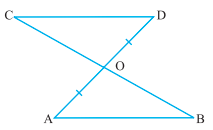Solution:

(i) Let us Consider Δ AOB and Δ DOC.

∠ ABO = ∠ DCO

(Alternate angles as AB || CD and BC is the transversal)

∠ AOB = ∠ DOC (Vertically opposite angles)

OA = OD (Given)

Therefore, ΔAOB ≅ ΔDOC (AAS rule)

1. In the given figure, E and F are respectively the mid-points of equal sides AB and AC of Δ ABC.

Show that BF = CE.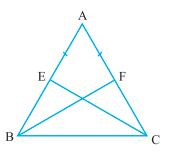Solution:

In Δ ABF and Δ ACE,

AB = AC (Given)
∠ A = ∠ A (Common)
AF = AE (Halves of equal sides)

So, Δ ABF Δ ACE (SAS rule)

Therefore, BF = CE (CPCT)